﻿ Internal Energy Functions of Thermodynamic SystemsPhysical Chemistry

p-ISSN: 2167-7042    e-ISSN: 2167-7069

2017;  7(3): 63-69

doi:10.5923/j.pc.20170703.02### Internal Energy Functions of Thermodynamic Systems

Xiaofu Wu

College of Environmental Science and Engineering, Central South University of Forestry and Technology, Changsha, P. R. China

Correspondence to: Xiaofu Wu, College of Environmental Science and Engineering, Central South University of Forestry and Technology, Changsha, P. R. China.
 Email: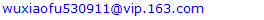Abstract

The present work presents a different angle to look into the concepts of internal energy functions of thermodynamic systems. The argument presented is that the total internal energy U of a thermodynamic system consists of two basic components, Uk, the kinetic energy of the material particles moving in the interior space of the system, and Um, the energy stored in other forms inside the materials contained by the system. Internal energy is matter-dependent and energy exchange is thus a process of matter exchange. The expression of U as a function of pressure P or temperature T given volume V does not take Um into account. The relation U=q-w is a path function for closed systems without matter exchange. Work (w) as a path function is not quantitatively related to the change in U as the work done by a system on its surroundings should be equal to the work done by the surroundings on the system. Heat (h) can be either absorbed or released, and heat transfer is a process of mass transfer. The connections of Uk and Um with enthalpy H, entropy S, Helmholtz free energy A and Gibbs free energy G are Uk=H=TS, Um=A=G and U=TS+G.

Keywords: Internal energy, Kinetic energy, Material energy, Enthalpy, Entropy, Free energy

Cite this paper: Xiaofu Wu, Internal Energy Functions of Thermodynamic Systems, Physical Chemistry, Vol. 7 No. 3, 2017, pp. 63-69. doi: 10.5923/j.pc.20170703.02.

### 1. Introduction

There have been difficulties to fully understand the concepts of enthalpy H and entropy S though they are generally acknowledged state functions of thermodynamic systems. As a matter of fact the concepts of H and S are not more abstract than that of a thermodynamic system. In textbooks [1-5] used in universities there is no concrete definition of a thermodynamic system concerning its volume V. For ideal gases, V does not appear to be a necessary variable since the total internal energy U depends only on temperature T, disregarding the change in V [1-3].
Both H and S are linked to heat uptake q and their incremental values can be determined, respectively, by ∆H=qP and ∆S=qr/T, where the subscripts P and r denote, respectively, constant pressure and reversible process. In later years the Helmholtz free energy A and Gibbs free energy G were further introduced as state functions based on their connections with, respectively, total and nonexpansion work [1-3]. The present study presents a different angle to look into the concepts of these traditionally defined state functions with focus on their heat and work related natures.

### 2. State Function and Path Function

The classic mathematical expression of the first law of thermodynamics is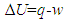(1)
The first law holds without doubt as energy can be neither created nor destroyed [1-5]. Thus Equation (1) appears to be a logical expression that the change in the total internal energy of the system ∆U would be equal to the energy gained by withdrawing heat q less the energy lost by doing work w. The relation ∆U=q-w, however, is a path function that could possibly be valid only for closed systems without matter exchange. In open systems there will be mass transfers and thus ∆Uq-w while in isolated systems, ∆U=0. Note that ∆U=0 in isolated systems is the basic algebraic definition of the first law of thermodynamics [2, 3]. It is known that w is a path-dependent variable and ∆U can correspond to numerous w values subject to the history of the process. Thus one cannot simply relate ∆U to w without specifying the condition. When P was kept constant, one might have ∆U=qP+w subject to w=PV, which would then give the change in enthalpy, ∆H=qP=∆U+PV [1-3]. It is noted that ∆H=∆U+PV is subject to five path conditions: the system withdraws heat (qP>0); the system does expansion work (wexpansion=PV); P remains constant (∆P=0); the composition and total molar quantity of the materials of the system are kept unchanged (∆n=0); and nonexpansion work is impossible (wnonexpansion=0). In any process other than this, for example, if wnonexpansion≠0, ∆H=qP≠∆U+PV.
The introduction of H presents a new expression for U, saying that U is the difference between H and PV. The generalized expression U=H-PV, however, is confusing. According to its concept, H should be a part of U. It would be unreasonable that H could be greater than U, and U be negatively related to PV since PV≥0.
Another expression for U given by H. L. P von Helmholtz [2, 3] is U=A+TS. The free energy theory splits U into two parts, A (the Helmholtz free energy) and TS, based on their connections with, respectively, work and heat. The term TS should then be a measure of heat since by definition, TS=qr and H should be in principle a function of S. Given ∆H=0 in isolated systems, ∆S≥0 according to the third law of thermodynamics, which denies the dependence of H on S.
Introduction of the Gibbs free energy G by J. W. Gibbs [1-5] gives new expressions not only for U but also for H, namely, U=G+TS-PV and H=G+TS. It sees that in addition to TS, which is linked to heat, G, which is linked to nonexpansion work, is also a component of H. This would suggest that H is not a state function uniquely associated with heat. This can be explained since the formulation of H is subject to a constraint that nonexpansion work is impossible. If the system does nonexpansion work (the net work related to the change in G), U should be further lowered such that the real enthalpy', H'=U+PV-G=H-G=G+TS-G=TS. Comparing U=(TS)+A with ∆U=q-w also shows that (TS) and A correspond, respectively, to q and -w. Thus if enthalpy is a heat-related state function, H should be equal to TS rather than to G+TS. As a matter of fact the generalized forms of H, A and G, as being clearly stated in their formulation processes, are all subject to path conditions given either P, V, T or n or a combination of them [1-3]. The application of them should thus be restricted, respectively, to their specified conditions.
The above discussed problems initiate from expressing U as a function of heat and work. The concepts of H, S, A and G are abstract because they are not linked to the origin or nature of internal energy possessed by a thermodynamic system. The state of a single-phase gas system with fixed composition can be characterized by any two of the three variables, P, T and V. It is then believed that for a system of fixed mass, U could be written in any of the three forms, U(T, V), U(P, V) or U(T, P) [2, 3]. When partial derivatives were used to describe the dependence of U on V and T or P, an infinitesimal change in U was given as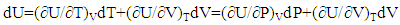(2)
However, when viewing it at a macroscopic level, one understands that the internal energy of a thermodynamic system can take on a number of forms such as the kinetic energy of the molecules; the potential energy of the constituents of the system; the energy related to molecular or atomic vibrations and rotations; and the energy stored in forms of chemical bonds, etc. It is known that for a gas system, its P and T are originated from the molecules' random motions and collisions, and they are thus the measures of the integrated impact or the kinetic energy of the moving molecules contained in the system. Given V and n, a gas system that have low T and P values can have a high U value if the gas molecules contain high amounts of internal energy in other forms than kinetic energy. If a gas molecule flows out from a system, the system will lose not only its impact on internal T and P but also its mass as an energy source while the related changes in T and P only account for its impact. The changes in T and P in isolated systems are apparently not related to the changes in U since in isolated systems, U=0. Therefore, even for a gas system of fixed mass, it would be inappropriate to write U as U(T, V) or U(P, V) because such expressions could not take into full account all forms of internal energy stored in the system. Since work and heat are related to, respectively, the two intensity factors, P and T, the validity of both Equations (1) and (2) needs to be re-examined.

### 3. Kinetic Energy and Material Energy

A molecule possesses an internal energy (or material energy) that is stored inside the molecule (Em). A moving molecule may also have a kinetic energy (Ek) relative to a static system. Therefore, the total energy related to a material consists of two basic energy components, Em and Ek. Using Ei to represent the total amount of energy that can be extracted from material i, we have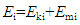Since a thermodynamic system is a system that contains the materials under study, the total internal energy of the system U should be equal to the sum of energy contributed by all materials contained in the system (Figure 1), namely,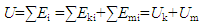(3)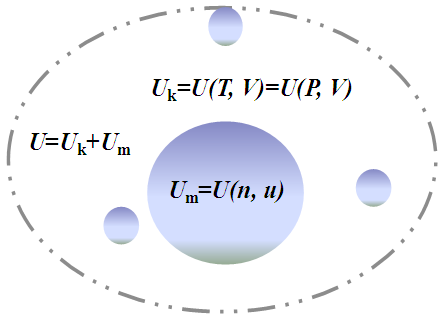Figure 1. Schematic of internal energy components: The total internal energy U=Uk+Um, where Uk, as a function of temperature T or pressure P given volume V, denotes the sum of the kinetic energy of the materials moving in the interior space of the system, and Um, as a function of mass quantity n and chemical potential μ, stands for the sum of the energy stored in other forms inside the materials contained by the system
In other words, one may say that if a material is removed from a system, the system loses its contribution to both Uk and Um.
The concepts of Uk and Um defined by Equation (3) can be applied to all types of systems at different macroscopic levels. For simplicity, for a system containing gas molecules only, Uk denotes the sum of the kinetic energy of the molecules moving in the interior space of the system while Um stands for the sum of the energy stored in other forms inside the molecules contained in the system. The term Um then consists of both the sum of the kinetic energy of the sub-particles (ions, atoms, etc.) moving in the interior space of the molecules and the sum of the energy stored in other forms inside the sub-particles contained by the molecules. As essential components of U, Uk and Um are properties of the system. They are thus state functions and have fixed values at a given system state.
The concept of Uk is of high significance as, in principle, Uk should be a function of T or P given V, or a function of V given T or P. We argue that it should be the Uk/V ratio rather than the U/V ratio that determines T and P of the system. For this reason, U in Equation (2) may have to be replaced by Uk simply because at a given state, Um has no direct impact on T and P. Though the nature of heat and the essential connection between T and P need to be further clarified in future studies, we can write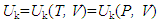(4)
To be more precise, for example, if molecular vibrations or rotations should also have an impact on P and T, Uk defined by Equation (4) should include that impact. By definition, Um should be related to the mass quantity and the nature of the materials contained in the system. Applying the concept of chemical potential μ defined by J. W. Gibbs and G. N. Lewis [1-3], we may write for a system given mass quantity n,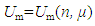(5)
Note that Um is independent of T, P and V, and if Umi of material i is not released, Umi has no impact on T and P. Transformation between Uk and Um can take place in thermodynamic systems. For isolated systems, ∆U=0, Equation (3) gives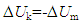(6)
Thus, given ∆U=0, we can have the connection between Uk and Um,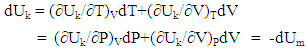(7)
It is important to note that Equations (6) and (7) hold as long as ∆U=0, which implies that the changes in system T, P or V or any combination of them are not necessarily related to the change in U of the system. In other words, the change in T, P or V may not always be a cause or a consequence of the change in U. Equations (6) and (7) obey the first law of thermodynamics.
It is necessary to mention that a thermodynamic system may also have a kinetic energy if the system is moving relative to its surroundings. The kinetic energy of the system, however, is not the internal energy of the system. Similar to that the kinetic energy of a molecule is a part of the internal energy of a system that contains the molecule, the kinetic energy of a system is a part of the internal energy of a larger system that contains the system. If the surroundings of a system stands for the rest of the universe, the kinetic energy of the system is then a part of the internal energy of the surroundings since the system can only move inside and have an impact on its surroundings. It is also necessary to mention that the kinetic energy of a molecule or system is not a property of the molecule or system. The speed of a molecule's or system's movement and related kinetic energies are relative magnitudes that cannot be determined without an external reference system. The concept of kinetic energy of a completely independent particle or an isolated system does not exist. In other words, such particle or system (for example, the universe) does not possess a kinetic energy.
Equation (3) shows an essential connection between energy and matter. If, based on Equation (3), a system contained no matter, both Uk and Um would be zero and the system would possess no energy. This would give a concept of empty system (or empty space) for, hypothetically, those systems containing no matter. Since a real empty system may not exist in the universe, a system that contains no matter may be referred to as an ideal empty system. It should be logic to think that since where there is no matter there is no energy, the change in the total internal energy of a system should always be associated with the change in its total mass quantity. This would imply that there should be no internal energy exchange if there were no matter flows across the boundary between the system and surroundings.
The Einstein mass energy equation defines a basic connection between energy E, mass M and light speed C,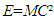which, since E=0 if M=0, gives support to the idea that energy is matter-dependent. Based on this equation, it would then be impossible to separate energy from matter. There should be no doubt that the exchange of Um between systems is a consequence of matter exchange. The momentum of a particle may be transferred to other particles in the same system by physical attraction or contact. The system will not lose its internal energy because of the particle attraction or contact in the system. The particles of a system do not contribute Uk to its surroundings unless they move out from the system to its surroundings. The concept of traditionally defined closed system infers the presence of such systems that allow energy exchange only. Assuming the presence of ideal empty systems, it would be difficult to imagine how energy could flow into a closed empty system that contained no matter.
It is not all materials contained in a system that can be exchanged under natural conditions. Small particles with high mobility can spontaneously move across the boundary between systems. Heavier materials may be held by gravity and remain static in the system. Such materials, if they do not emit heat, have no direct impact on system T and P except for occupying a portion of system space. Heavy materials do not spontaneously undergo matter exchange process unless additional forces are provided. Another important factor for matter exchange is the nature of the system wall. The concepts of open, closed and isolated systems should be linked to the their degrees of openness including permeability and conductivity regarding matter exchanges. Thus, spontaneous energy exchange should depend on two necessary conditions, the mobility of the materials and the degree of openness of the system. The traditionally defined closed systems that allow energy exchange only do not exist in reality.

### 4. Work and Heat

#### 4.1. Work Linked to the Change in V given P

Assuming that a work done by a system on its surroundings had led to a decrease in U of the system from Ui to Uf, and that in the process there had been no heat exchange, q=0, from Equation (1), we would have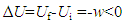Since ∆U had a fixed value which was equal to Uf minus Ui, the relation ∆U=-w would mean that given q=0, w could also be uniquely determined by the difference between Uf and Ui. Since, however, w is a path-dependent variable which cannot be uniquely determined by the difference between Uf and Ui, the conclusion that ∆U=-w given q=0 may not hold in general.
As a path function, the expansion work w is related to the change in V since w=0 if ∆V=0. Like w, the change in V or U is not a system property either because a system does not have a ∆V or ∆U value at a given state. The difference between w and ∆U is that w varies with path conditions while ∆U is always equal to Uf minus Ui no matter how much work has been done in the process. Systems can do work while the work performed may not be equal to the energy consumed. It has been understood that the change in U of a system could only be fully converted to work in reversible processes. If this were true, we would have w<-∆U since all natural processes are irreversible.
It has been generally believed that a work done by a system on its surroundings would lead to a decrease in U of the system associated with an equal increase in U of the surroundings, or vice visa. It is known that the performance of a mechanical work involves both acting and reacting forces. As a general rule, whatever the types of forces are, the acting and reacting forces (as vectors) will always be equal, appearing simultaneously but towards opposite directions. Thus in principle, any mechanical work (e.g., an expansion work) done by a system on its surroundings should be equal to the work done by the surroundings on the system. It is impossible to compress a system by a force unless the system is held tightly by a counterforce. Otherwise, the system will obtain an acceleration and move towards the direction of the force. Assume that a gas cylinder held by its gravity on the ground was compressed by an external force, and according to the traditional theory, the system should gain energy, namely ∆Usys>0. If the cylinder was an open system (Figure 2a), the increase in internal P due to the compression would lead to a gas flow out of the system; while if the cylinder was a traditionally defined closed system (Figure 2b), the increase in internal T due to the compression would lead to a heat flow out of the system. In both cases, the consequence would be ∆Usys=-∆Usur<0, meaning that the system would lose rather than gain energy because of the work done by its surroundings. If the cylinder was isolated to prevent matter and heat exchange (Figure 2c), the compression work (wsur) done by the external force should be equal to the work (wsys) done by the internal pressure to resist the compression, namely, wsur=PexternalV=-wsys or wsur+wsys=0. Assuming that the internal energy could be converted to "work flows", the amount of "work flows" flowed from the surroundings into the cylinder would be equal to that from the cylinder to the surroundings. Thus in accordance with the first law of thermodynamics that ∆U =0 in isolated systems, the energy exchange between the cylinder and its surroundings would eventually be zero, namely, ∆Usys=-∆Usur =0. The cases shown in Figure 2 indicate that mechanical work can only cause energy exchange between open and closed systems while the direction of the energy flow is not necessarily determined by the work but by the second law of thermodynamics.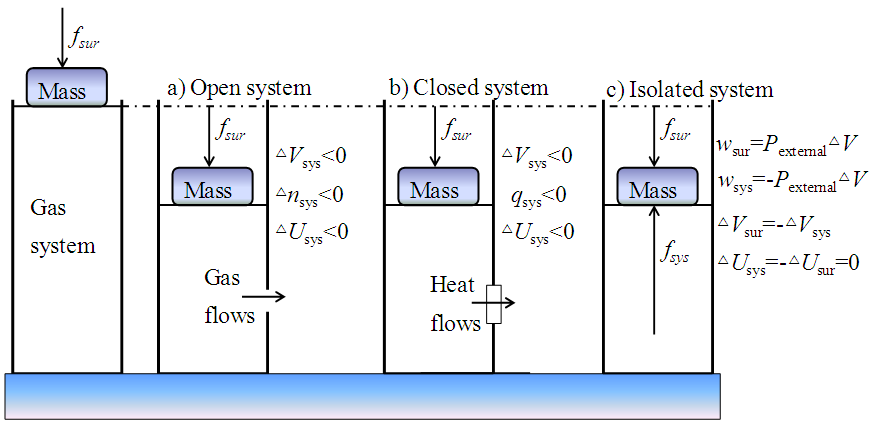Figure 2. Compression of a cylinder consisting of gases by an external force from the surroundings: a) Open system: ∆Usys <0 due to matter exchange; b) Closed system: ∆Usys <0 due to heat exchange; and c) ∆Usys=-∆Usur=0, and the work (wsur) done by the external force was equal to the work (wsys) done by the internal pressure to resist the compression, wsur=Pexternal∆V=-wsys
Work is not a system property while energy is. In other words, work is not a form of energy and thus energy cannot be transformed or converted into work. There should be no such concept of work flows. Energy can be transformed or transferred by work. After the work is done, the transformed or transferred energy remains either in other forms or in other systems. The concept of pressure is different from that of force. In order to have a pressure on a particle, there have to be at least two forces acting simultaneously on the particle in reverse directions. When an amount of energy is released, it generates forces towards all directions while the work under concern accounts only for a partial effect of the released energy. Work can be both an effect and a cause of energy exchange. If, for example, a mechanical work done by System A on System B had led to a break of System B, which caused a matter exchange between B and the surroundings of B, the change in U of B is not related to the work done by A but dependent on the difference in P between B and its surroundings. It is known that a small amount of work may initiate a series of chain reactions that can release large quantities of energy. In all such cases, the change in U is not quantitatively related to w, namely, ∆U≠-w.
Assume that there were three isolated systems A, B and C (Figure 3). Systems A and B were ideal empty systems with rigid walls and different volumes (VA and VB), and System C contained a single pure gas. Further assume that we were able to transfer simultaneously the same amount of gas from C to, respectively, A and B (Figure 3a), the total internal energy of C would decrease, ∆UC<0, associated with decreases in TC and PC due to the matter output. Since the amount of gases transferred to A is the same as that to B, nA=nB, and the transfer was carried out under the same condition, the amount of internal energy transferred from C to A should be equal to that to B. At the initial state, the internal energy was zero in both A and B, the total internal energy of A and B after the transfer should be equal, namely, UA=UB and ∆UC=-(UA+UB). Since nA=nB, if VA<VB, we have PA>PB, TA>TB and nA/VA>nB/VB, showing that UA and UB are uniquely related to the transferred mass quantity n and independent of P, T and V.
Now assume that we could manage to connect A and B in the following two different ways (Figure 3b): (I) using an open wall that allows gas molecules to diffuse freely between A and B, and (II) using a movable wall that allows system volume to expand freely between A and B. In accordance with the first and second laws of thermodynamics, the expected results would be:
Case I (open wall, Figure 3b-I): Gas molecules would diffuse from A to B until PA=PB, TA=TB and nA/VA=nB/VB. At the final state, since VA<VB, we would have nA<nB and UA<UB, showing that the matter exchange had led to a decrease in UA and an increase in UB, and thus ∆UA=-∆UB<0.
Case II (movable wall, Figure 3b-II): The volume of system A would expand until PA=PB, TA=TB, VA=VB and nA/VA=nB/VB. At the final state, we would still have nA=nB and UA=UB, showing that the expansion work had not caused any change in UA and UB, and thus ∆UA=∆UB=0.
The expected results in Cases I and II support the argument that work does not transfer internal energy between systems unless matter exchange takes place. In Case I, the difference in P between Systems A and B before reaching equilibrium also did a mechanical work that pushed the gas molecules to move from A to B. Since it was the difference in P or Uk/V that did the work, w should be only related to the change in Uk, namely, w-∆Uk. Because of the work, System A lost both the mass and impact of the molecules diffused to System B, and the consequences would be ∆Uk,A<0 and ∆Um,A<0. We should thus write w≠-∆UA since ∆UA = ∆Uk, A + ∆Um, A.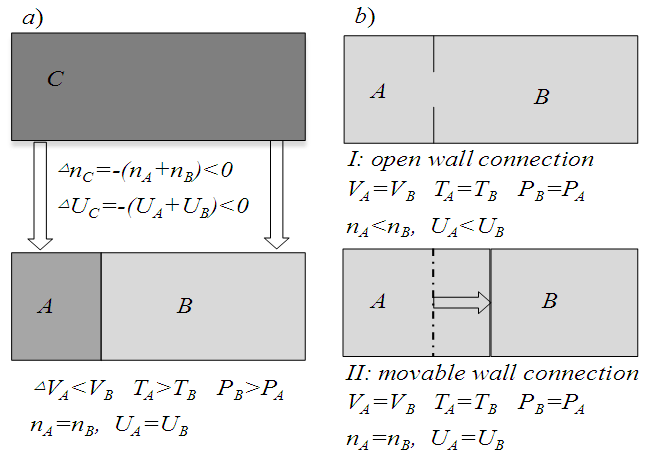Figure 3. Matter and energy exchanges in Systems A, B and C: Systems A and B are ideal empty systems with rigid walls, and C contains a single pure gas: a) The final states after transferring simultaneously the same amount of gas from C to, respectively, A and B; b) The finial states after connecting A and B by (I) an open wall to allow free diffusion and (II) a movable wall to allow free expansion
Systems A and B contained only gas molecules with high mobility. When systems A and B became fully open to each other (Figure 3b-I), they were actually merged into one system. The work done on the molecules had not caused any change in but just evened the distribution of U of the merged system. As in the merged system the work done by molecule i on molecule j is equal to the work done by j on i, wi+wj=0, we have ∑wi=0. The conclusion ∑wi=0 may give a logical support to the first law of thermodynamics, namely that ∆U=0 in isolated systems because ∑wi=0. The relation ∑wi=0 should hold in general for all kinds of work in all types of systems. For an example, generation of an electric power in a system is a process of increase in Uk/V (not necessarily U/V) while the system will not lose its internal energy if its generated electricity is not used. Electric work involves conduction of electric current which is a flow of electrons and thus electric work is associated with matter exchange.

#### 4.2. Heat Related to T Given V or S

The argument presented here is that all materials and systems have heat states that can be uniquely determined by T given V or S, heat can be exchanged, and heat is thus a state function. Assuming that heat exchange had led to an increase in U of a system from Ui to Uf, from Equation (1) given in the process w=0, we would have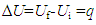showing that given w=0, the uptake of heat q can be uniquely determined by the difference between Uf and Ui. This, in accordance with the first law of thermodynamics, would lead to a conclusion that if heat exchange did cause changes in U, heat should be a state function related to U. There appears to have no conclusive evidences to indicate that heat is a path function. The unique reasoning on this matter noticed in textbooks [2, 3] was based on the relation ∆U=q-w, saying that because given the initial and final states, ∆U is the same for each path, w can be different for each path, and q has to be different for each path. This conclusion would lack a logic base if ∆Uq-w.
The concept of q is different from that of w. The symbol w denotes the work done by the system while q does not denote the heat but the uptake of heat by or, equivalently, the change in heat state of the system. A system can do but cannot have (or possess) a work. In contrast, a system can either withdraw or release heat, implying that a system can either obtain, or lose, or possess heat. Fuel materials disappear when being burned to generate heat, indicating that heat exchange involves matter exchange. Experiences tell us that heat can be directly transferred by mass transfer or, similar to electricity, by conduction. Like electric current, the conducted heat should also be a current of hot particles. Similar to other radiation activities, heat emission is a matter exchange phenomenon. It is thus puzzled why heat has been treated as a path variable in thermodynamics that deals with thermal phenomena.
The quantity of heat withdrawn by a system in a reversible process is given by qr=TS. Since S has been proved to be a system property, TS is a state function. As a matter of fact, if in an energy exchange process nothing else but heat exchange takes place, following the law of energy conservation, we should always have q=∆(TS) disregarding the reversibility of the process. The traditionally defined thermal equilibrium is actually determined by the Uk/V ratio, namely, systems A and B reaching thermal equilibrium at TA=TB corresponding to UkA/VA=UkB/VB. This implies that at the equilibrium state, systems A and B can have different Um/V and thus U/V values. Since TS is a function of T determined by Uk/V, from Equation (4), we can establish the connection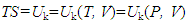(8)
which gives q=∆(TS)=∆Uk≠∆U. This, in accordance with Equations (6) and (7), explains why the changes in T, P or V of a system are not necessarily related to the change in U of the system. The above discussions support the argument that both Equations (1) and (2) do not hold in general.

### 5.Connections with Conventional State Functions

Equation (8) shows a connection between VP and TS, inferring that the energy term VP is another expression of TS. Before establishing this connection, however, the fact that PV is not a component of the traditionally defined H and A needs to be clarified. From ∆H=∆U+PV and ∆A=∆G-PV, we see that for systems with rigid walls, ∆V=0, ∆H=∆U and ∆A=∆G, suggesting that PV is, at least, not an essential component of the traditionally defined H and A.
Recalling the path conditions for ∆H=qP=∆U+PV, we could argue that for a system to expand under a constant pressure condition, energy must be provided from somewhere to do the expansion work. Otherwise, it would violate both the first and second laws. The system withdrawn heat (qP>0) and thus no heat flowed out of the system. Since the system did not get any energy from matter exchange (∆n=0) and nonexpansion work (wnonexpansion=0), the only possibility left for the system to maintain constant P (and thus Uk/V) while expanding would have to use the heat obtained from its surroundings. Then we would have PV=qP. Since qP>0, ∆U>0, which should in turn cause changes in the state of at least one of the system properties. In other words, the inputted heat had to be deposited somewhere in the system. Since only V of the system changed, this change had to be an effect of the heat uptake. Note that PV>0 and PV is an energy term, meaning that the system gained rather than lost energy. In the process the surroundings lost an equal amount of heat, which could be accounted for by its reduced space volume, ∆Usur=-qP=-PV. Thus for the system, ∆Usys=∆H=qP=PV, implying that the expansion was a consequence of heat uptake. In other words, the system did not lose energy because of the expansion work.
Given ∆H=0, the relation H=U+PV=G+TS leads to two seemingly related consequences: ∆U=-∆(PV) and ∆G=-∆(TS). This means that if H remains constant, the change in G will only cause the change in TS while the change in U will be uniquely determined by the change in PV. The consequence ∆U=-∆(PV) can hardly be true since there is no reason why U should decrease with increasing VP. The explanation is simple because ∆H=0 does not satisfy the basal condition for generalizing H, which demands, at least, ∆H=qP≠0. Despite of this, since G is a component of U, and changes in G may lead to changes in U, there had to be a connection between them and the possible link could be ∆(TS)=∆(PV). This link sounds reasonable as it confirms the connection between TS and PV. Given ∆H=0 in isolated systems, however, if ∆(TS)=∆(PV), ∆G=-∆(TS)=0 since ∆U=-∆(VP)=0, while if ∆(TS)=∆(PV)≠0, ∆U≠0 unless ∆U≠∆H-∆(PV). The relation ∆G=-∆(TS) given ∆H=0 defines a specific condition under which the change in G can be fully converted to that in TS in the system, implying that the change in G causes no changes in U. In other words, the precondition for ∆G=-∆(TS) is ∆U=0, meaning that ∆H=0 is a synonym of ∆U=0. Then, giving ∆H=0, ∆U=0, ∆G=-∆(TS) and ∆A=∆U-∆(TS)=-∆(TS)=∆G.
The connection between P and T given by the empirical relation (or the ideal gas law) [1-3] is VP=nRT, where R is the gas constant. The ideal gas law gives a logical support to the connection between PV and TS. The total system volume V is equal to the space unoccupied by materials Vk plus that occupied by materials Vm. Let Vk/V+Vm/V=δ+(1-δ), which gives Vk=δV. We can argue that it should be VkP rather than VP that is proportional to nRT, namely,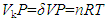which suggests that the empirical relation VP=nRT may only hold at δ≈1 and VkV when n approaches zero. This can be a logical explanation to the observed phenomena that the ideal gas law did not work satisfactorily at high P due to increasing n. Based on Equation (8), for unifying the unit, we can use a proportional factor k to connect δVP and TS,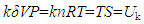(9)
which in turn gives S=k'V=k''n, suggesting that S is a capacity factor related to V given P or n given T.
Since VP is no longer a component of the traditionally defined A and H, we have A=G, H=TS and U=H+A. The Gibbs free given by J. W. Gibbs and G. N. Lewis is expressed as G=∑niμi. From Equation (5) we see that G stands for the internal energy stored inside the materials, namely, G=Um. The connections of U, Uk and Um with H, A, G and S are thus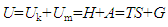(10)
The conventional concepts of enthalpy, entropy and free energy remain valid in Equation (10) except for being less abstract. A thermodynamic system consists of six basic properties, n, V, S, μ, P and T. For a system containing a single pure material at a given state, Equation (10) defines the three intensive properties, respectively, as μ=Um/n, P=Uk/Vk and T=Uk/S.

### 6. Conclusions

The relation U=TS+G may be considered the simplest expression of U at the highest macroscopic level. Depending on the interest of study, both G and TS can be divided into detailed portions in accordance with the nature of materials and systems. It is necessary to mention that the present work agrees with the existing laws and experimental findings except for presenting different interpretations of the internal energy functions. The following conclusions can be drawn from the above discussions:
(1) The total internal energy U consists of two basic components, Uk, the total kinetic energy of the material particles moving in the interior space of the system, and Um, the total internal energy stored in other forms inside the materials contained by the system.
(2) Energy is matter-dependent and energy exchange is a process of matter exchange.
(3) Work as a path function is not quantitatively related to ∆U.
(4) Heat is a state function that can be determined by T given V or S.
(5) Components Uk and Um are connected to H, S, A and G as Uk=TS=H and Um=A=G.

### ACKNOWLEDGMENTS

The present study forms part of the projects (2012GS430203, 2014BAC09B00) supported by the Ministry of Science and Technology, China, and the Educational Department of Hunan Province (the Key Subject and Lab).

### References

  Adam, N. K. (1956). Physical Chemistry. Oxford University Press, Amen House, London, UK, p. 250-300.  Engel, T. and Reid, P. (2006). Physical Chemistry. Pearson Education, Inc., Benjamin Cummings, New York, USA, p. 2-4, 13-30, 44-47, 85-90, 113-122, 6.  Fu, X.C., Sheng, W. X. and Yao, T. Y. (1990). Physical Chemistry. High Education Press, Beijing, p. 13-174.  Brown, T. L., LeMay Jr, E. H., Bursten, B. E. and Murphy, C. J. (2006). Chemistry the Central Science. Tenth edition. Pearson Education, Ltd., London, UK, p. 167-196; 803-845.  Kondepudi, D. and Prigogine, I. (1998). Modern thermodynamics: from heat engines to dissipative structures. John Wiley & Sons Ltd. Chichester, England. p. 31-56; 123-141; 153-189.